• 本程序是短时傅里叶变换matlab程序，短时傅里叶变换是信号分析的重要手段，在视频，图像，音频信号中有着非常重要的作用。
• 短时傅里叶变换（STFT）是和傅里叶变换相关的一种数学变换，用以确定时变信号其局部区域正弦波的频率与相位。
• 时频分析 短时傅里叶变换 Matlab程序 好用 地震信号处理
• 参数可自由选择，可以根据自己需要选择步长、窗函数种类从而实现短时傅里叶变换
• 通过汉明窗将一串信号进行分解得到不同频率的信号，合成信号验证，主要是在MATLAB中实现，DTFT，可通过控制窗函数的长度和移动幅度来控制频率的分段
• MATLAB代码，能够实现短时傅里叶变换。可以直接用来处理数据。STFT
• 参数可自由选择，可以根据自己需要选择步长、窗函数种类从而实现短时傅里叶变换。 参数可自由选择，可以根据自己需要选择步长、窗函数种类从而实现短时傅里叶变换
• 读取TXT格式数据文件，进行短时傅里叶变换，并将结果保存为图片的MATLAB脚本。短时傅里叶变换是一种经典的时频分析算法，常用于故障诊断等信号处理与分析领域。
• 短时傅里叶变换MATLAB实现代码，有效完成时频分析
• 短时傅里叶变换matlab程序 function [Spec,Freq]=STFT(Sig,nLevel,WinLen,SampFreq) %计算离散信号的短时傅里叶变换 % Sig 待分析信号 % nLevel 频率轴长度划分默认值512 % WinLen 汉宁窗长度默认值 64 % SampFreq ...
• 对信号进行短时傅里叶变换和小波变换，观察其时频特性，压缩包中含有需要用到的测试信号文件，可以直接运行，供参考
• matlab时频分析工具箱最新版 1.把工具箱解压到一个地方 2.打开matlab 3.File -> Set Path... -> Add with Subfolders... ->选择刚才解压的文件夹 这样就添加完成了，你就可以调用工具箱中的函数了
• 对一段音频信号进行短时傅里叶变换（或小波变换matlab源码），处理得到含频率和时间成分的语谱图（matlab+python源码）
• matlab时频分析之短时傅里叶变换 spectrogram 短时傅里叶变换常用于缓慢时变信号的频谱分析，可以观察沿时间变化的频谱信号。 其优点如下图所示，弥补了频谱分析中不能观察时间的缺点，也弥补了时域分析不能获取频率...
matlab时频分析之短时傅里叶变换 spectrogram
短时傅里叶变换常用于缓慢时变信号的频谱分析，可以观察沿时间变化的频谱信号。
其优点如下图所示，弥补了频谱分析中不能观察时间的缺点，也弥补了时域分析不能获取频率的缺点。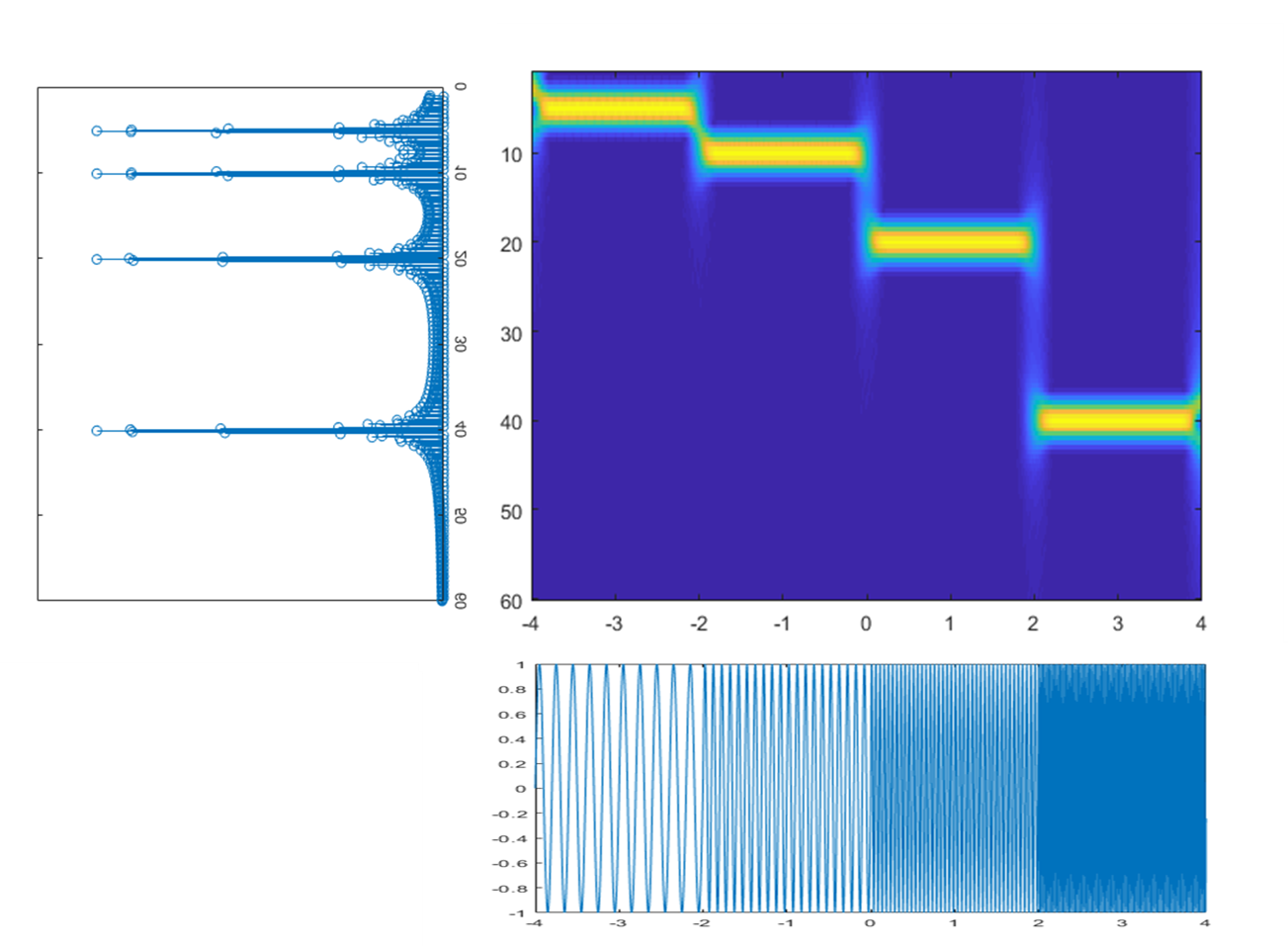1 STFT的基本原理
基本原理可以理解为对一段长信号，截取每一段时间的短信号做fft，将得到的频谱图时间沿时间轴排列，及可得到时频的云图。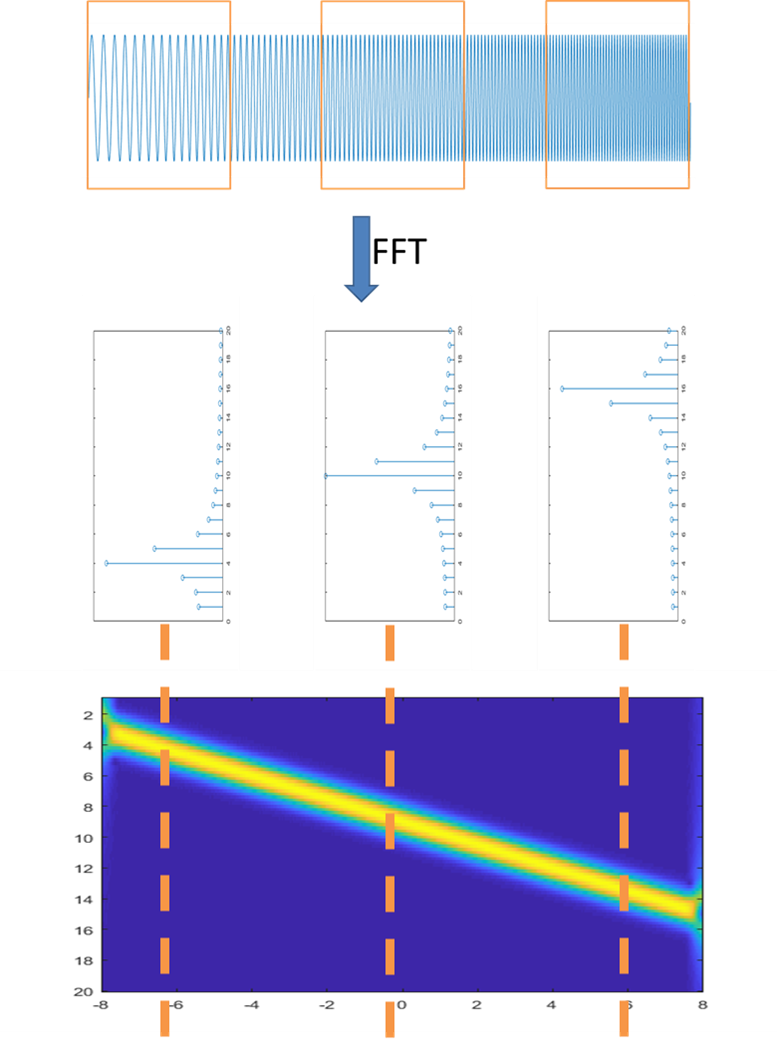2 matlab中实现
这里采用最基础的spectrogram函数作为分析函数。虽然在其它很多地方都能找到能实现更高级用法的函数，比如逆变换： https://ww2.mathworks.cn/matlabcentral/fileexchange/45577-inverse-short-time-fourier-transform-istft-with-matlab 但是这里只是单纯的做可视化分析。
代码如下：
%短时傅里叶变换展示
fs=2^10;    %采样频率 fs=65536hz
dt=1/fs;    %时间精度
timestart=-4;
timeend=4;
t=(0:(timeend-timestart)/dt-1)*dt+timestart;
L=length(t);

%设置信号
z=sin(2*pi*5.*t).*(t<-2)+sin(2*pi*10.*t).*(t>=-2&t<0)+...
sin(2*pi*20.*t).*(t>=0&t<2)+sin(2*pi*40.*t).*(t>=2);
z2=wextend(1,'sym',z,round(length(z)/2));%镜像延拓

wlen=512;%设置窗口长度。窗口越长时间分辨率越差，频率分辨率越好。
hop=1;%每次平移的步长，最小为1。越小图像时间精度越好，但计算量大。
z2=wkeep1(z2,L+1*wlen);%中间截断

%做短时傅里叶
h=hamming(wlen);%设置海明窗的窗长
f=1:0.5:60;%设置频率刻度

[tfr2,f,t2]=spectrogram(z2,h,wlen-hop,f,fs);
tfr2=tfr2*2/wlen*2;
figure
imagesc(t2+timestart-wlen/fs/2,f,abs(tfr2))

3 窗口长度与分辨率
短时傅里叶变换的分辨率和窗口长度wlen的选取，频率的刻度f、平移的步长hop都有关系。
spectrogram函数可以选取较密的频率刻度来增加频域的分辨率，减小平移步长增加时间的分辨率。
但是对于短时傅里叶变换，最重要的还是窗口长度的选取。当频域刻度和平移步长足够密时，增加的只是生成图像的大小，但是物理层面的分辨率却没有改变。改变物理层面分辨率的就是窗口长度。
下图为同一个信号，选取不同窗口长度的图像。其余量不变。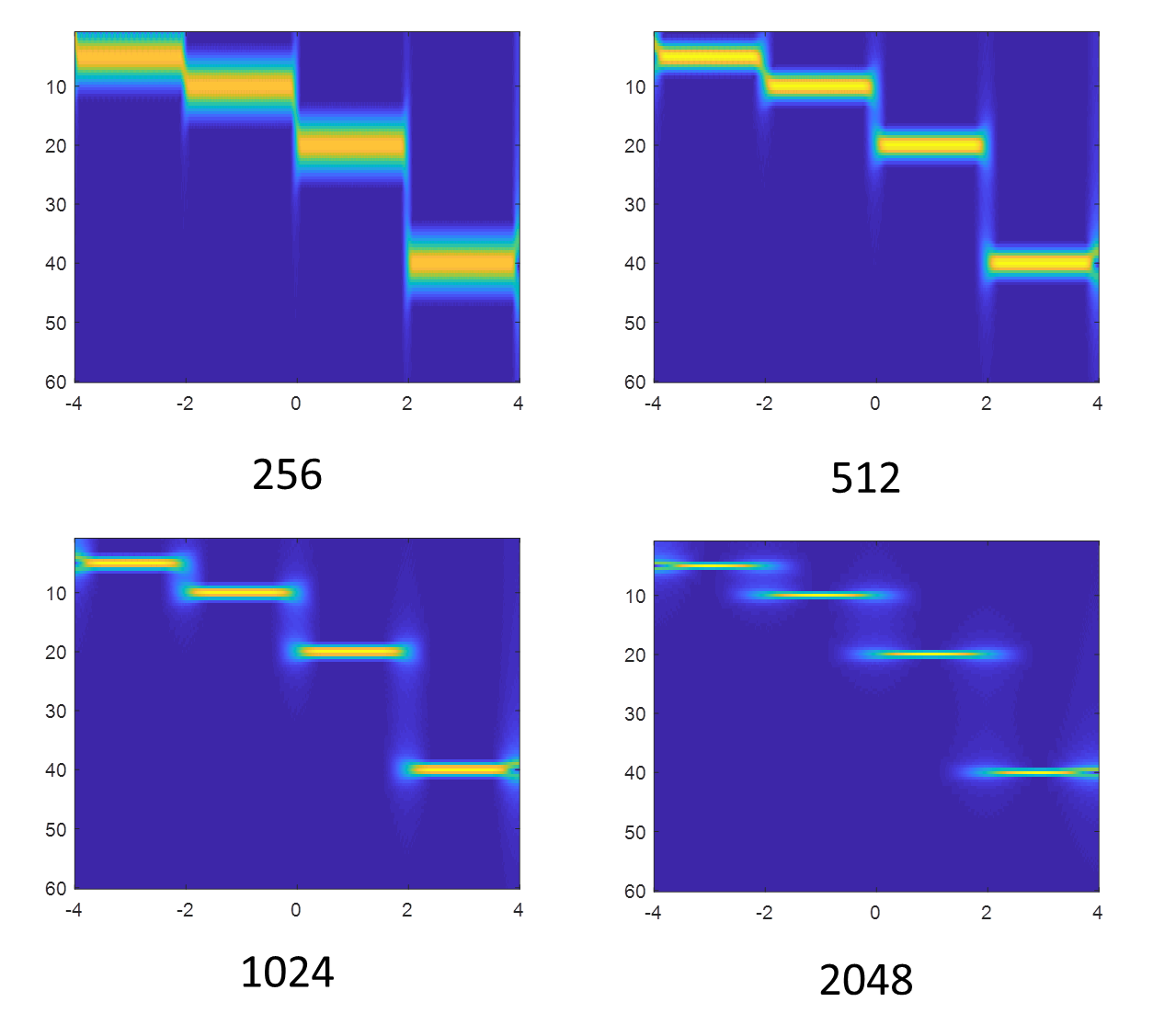窗口长度大的话，比如2048，频率能够清晰的显示出来，但是时间上有些模糊。窗口长度比较小比如256，时间上的分界线能够清晰显示，但是频率的值却不能清晰读出。
这就是窗口的影响。可以想象，当窗口大到整个信号长度时，短时傅里叶变换就退化为傅里叶变换，没有时间维度。当窗口小到单个采样点时，分析也就退化为时域分析，无法做频域分析。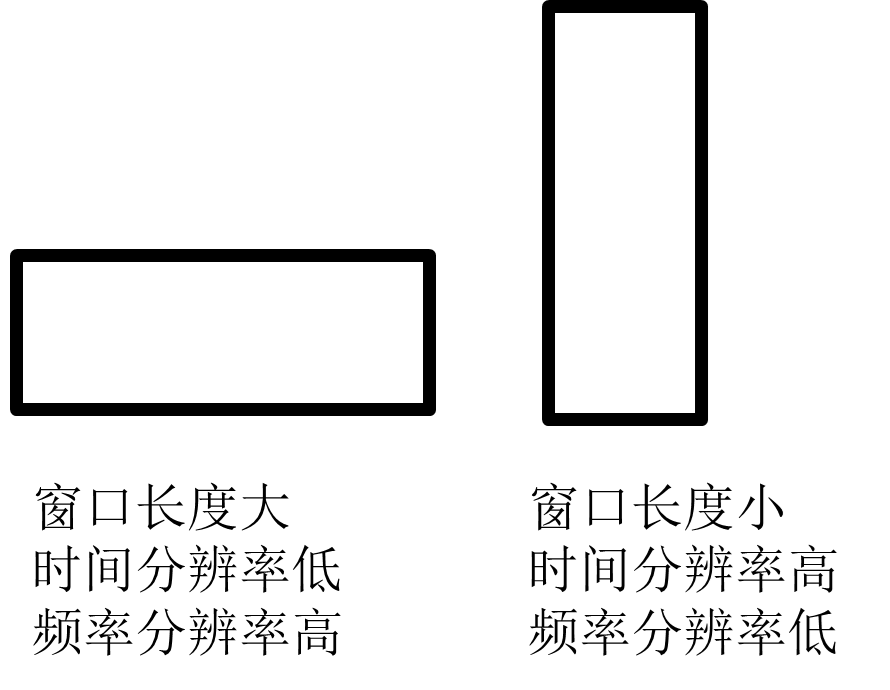4 提高频率分辨率的方法
matlab中的spectrogram函数自带两种提高频率分辨率的方法。
第一种是利用功率谱，将能量集中的原理，提高频率分辨率。 用法为： spectrogram(x,window,noverlap,f,fs,‘reassigned’,‘yaxis’); 前面的同第2节例子，在后面增加了’reassigned’选项。但是这个选项似乎不能用作输出，只能单独以图像形式显示，不知道为什么。
下图为窗函数为256时的两个对比。可以看到右边图的频率分辨率相比左图有了较大提升。但是存在许多噪点。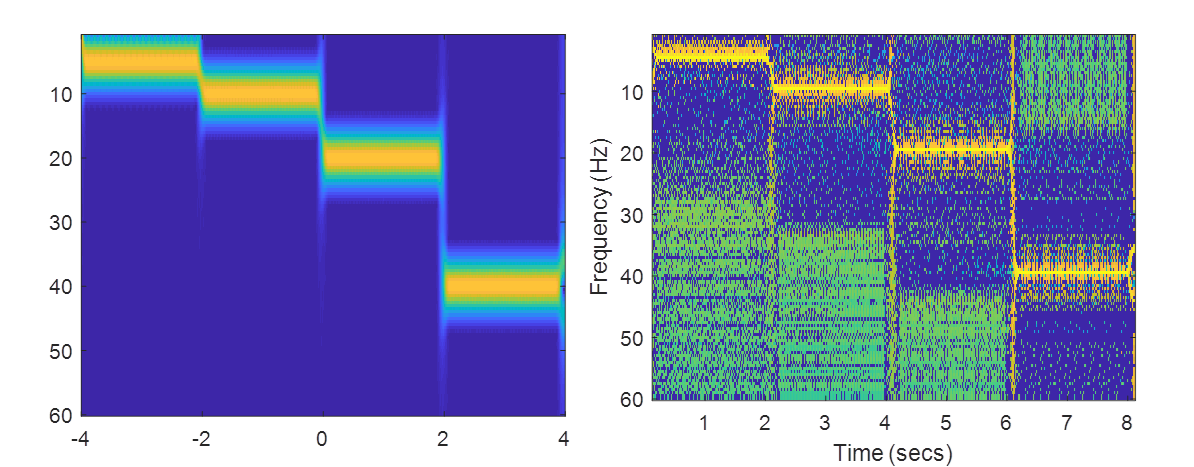第二种是将小于某个数值的数归零，提高频率分辨率。 格式如下： spectrogram(___,‘MinThreshold’,thresh) 参考第2节中的格式如下 spectrogram(z2,h,wlen-hop,f,fs,‘MinThreshold’,-14,‘yaxis’); set(gca,‘YDir’,‘reverse’); 结果如下图所示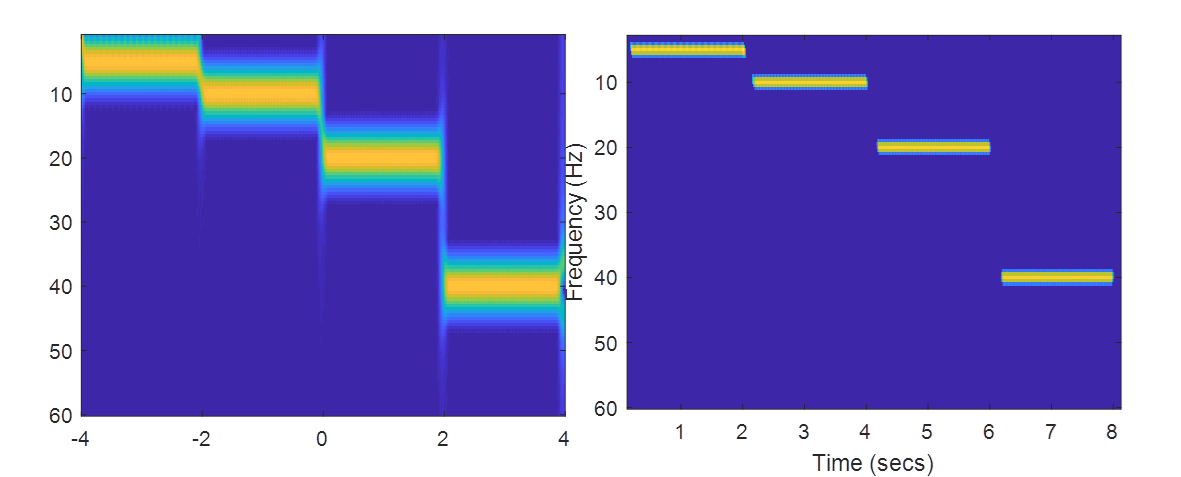5 提取相位信号
短时傅里叶变换不仅可以提取信号的幅值，还可以提取信号的相位。 幅值的提取方法为abs，相位的提取方法为angle。
例：
clear

z=mtlb;
L=length(z);
t=(1:L)/Fs;

z2=wextend(1,'sym',z,round(length(z)/2));
wlen=512;%设置窗长
hop=1;%平移步长
z2=wkeep1(z2,L+1*wlen);%中间截断

h=hamming(wlen);%设置海明窗的窗长
f=100:1:1000;
[tfr2,f,t2]=spectrogram(z2,h,wlen-hop,f,Fs,'reassigned','yaxis');
tfr2=tfr2*2/wlen*2;
figure
imagesc(t,f,angle(tfr2))%提取相位
colormap([linspace(0,1,32)'*ones(1,3);linspace(1,0,32)'*ones(1,3)])

信号的相位图如下：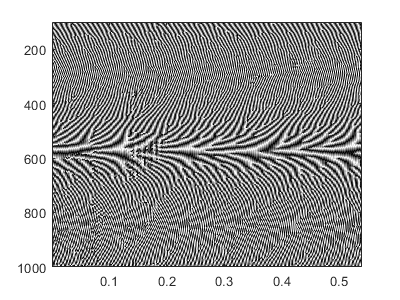展开全文STFT 时频分析 信号处理
• 短时傅里叶变换matlab程序,快速傅里叶变换及其应用matlab,matlab源码
• 短时傅里叶变换 matlab源程序，供大家参考学习
• 短时傅里叶变换（stft）的Matlab源码，已封装为Function函数，可直接根据源码注释调用
• 产生线性调频信号，联合分析方法，其思想是假定信号在较的时间内是平稳的，它将一个的变化的信号分为若干个时间段，在每个时间段内计算信号频谱，然后将各个时间段内信号频谱堆叠显示，从而了解信号频率成分随时间...
• 包含 4种 分数傅里叶变换matlab代码实现方式，可以用于图像加密，分数傅里叶变换分析
• 班级 实验地点 实验日期 实验序号1 实验类型验证性 学时6 一实验项目名称用matlab计算离散短时傅里叶变换 二实验目的与要求 用matlab计算傅里叶变换傅里叶变换 三实验内容 1.求正弦信号f(t)=ejw0t的短时傅里叶...
• 实现FFT点数可调、步进可调的短时傅里叶变换；实现加窗功能，窗类型可选。 代码如下 Fs=1000; %采样率1KHz Ts=1/Fs; %点时间间隔0.001s L=2048; %2048点数 t=(0:L-1)Ts; %采的时间为20480.001=2.048s %t1=(0:L/4-13)...
实现FFT点数可调、步进可调的短时傅里叶变换；实现加窗功能，窗类型可选。 代码如下 Fs=1000; %采样率1KHz Ts=1/Fs; %点时间间隔0.001s L=2048; %2048点数 t=(0:L-1)Ts; %采的时间为20480.001=2.048s %t1=(0:L/4-13)Ts; %500个点 %t2=(0:L/4+35)Ts; %548个点 t1=(0:L/4-13)Ts; %512-13=499;500个点 t2=(0:L/4+35)Ts; %512+35=547；548个点 %y=sin(502pit)+1; %信号周期:T1=50Hz，T2=120Hz;幅度范围为-2~2; %y=sin(502pit)+sin(1202pit)+2; %输出无符号十进制,范围0~4 %x = [2cos(2 * pi * 10 * t1+pi/2),2cos(2 * pi * 20 * t1+pi/2),2cos(2 * pi * 30 * t1+pi/2),2cos(2 * pi * 40 * t1+pi/2)]+2;%输出无符号十进制,范围0~4；2048个点 x = [2sin(2 * pi * 10 * t1),2sin(2 * pi * 20 * t1),2sin(2 * pi * 30 * t1),2sin(2 * pi * 40 * t2)]+2; y = x1023/8192; sinwave=round((y2^12));%输出有符号十进制数据；12位数据，最大2047；幅度-2~2 %%%%%%%%%%循环FFT PointNum=1024; Steps=256; Times=5; Waveform_Original_Record=zeros(1,TimesPointNum); Waveform_Windowing_Record=zeros(1,TimesPointNum); fft_phase_arranged=zeros(1,TimesPointNum); fftr_amplitude_arranged=zeros(1,TimesPointNum); f=zeros(1,TimesPointNum); %加窗处理 Hanning = hann(PointNum,‘symmetric’); Hs_Trans=[Hanning.’ Hanning.’]; %r1=round(Hs_Trans.sinwave); for iteration = 0:1:Times-1 Waveform_Original_Record(1,iterationPointNum+1:iterationPointNum+PointNum)=sinwave(1,iterationSteps+1:iterationSteps+PointNum); Waveform_Windowing=(Hanning.’).sinwave(1,iterationSteps+1:iterationSteps+PointNum); Waveform_Windowing_Record(1,iterationPointNum+1:iterationPointNum+PointNum)=Waveform_Windowing; fft_result=fft(Waveform_Windowing); %fft运算 fft_phase=angle(fft_result); fft_phase_arranged(1,iterationPointNum+1:iterationPointNum+PointNum)=[fft_phase(PointNum/2+1:PointNum) fft_phase(1:PointNum/2)]; fftr_amplitude=abs(fft_result/PointNum); %幅度归一，双边累加 fftr_amplitude_arranged(1,iterationPointNum+1:iterationPointNum+PointNum)=[fftr_amplitude(PointNum/2+1:PointNum) fftr_amplitude(1:PointNum/2)]; %取正半边 %f_pos=(1:L/4)Fs/(L/2); %f_inv=-1fliplr(f_pos); %f=[f_inv f_pos]; f_pos=(1:PointNum/2); f_inv=-1fliplr(f_pos); f(1,iterationPointNum+1:iterationPointNum+PointNum)=[f_inv f_pos]+PointNum/2+iterationPointNum; end %%%%%%%%%单次FFT % a=7; % r1=(Hs.’).sinwave(1,a128+1:a128+1024); % fftr=fft(r1); %fft运算 % fftr_normalized=fftr/1024; % fft_divide=angle(fftr); % fft_phase=[fft_divide(L/4+1:L/2) fft_divide(1:L/4)]; % %fftr_mod=2abs(fftr2/L); %幅度归一，双边累加 % fftr_mod=2abs(fftr2/L); %幅度归一，双边累加 % fft_pos=[fftr_mod(L/4+1:L/2) fftr_mod(1:L/4)]; %取正半边 % %f_pos=(1:L/4)Fs/(L/2); % %f_inv=-1fliplr(f_pos); % %f=[f_inv f_pos]; % f_pos=(1:L/4); % f_inv=-1fliplr(f_pos); % f=[f_inv f_pos]; %%%%%%%%%%%%%计算公式 %f=(1:L/4)Fs/(L/2); %频率信息解析,此时步进为Fs/(L/2),点数为L/4。 %r=ceil(y(2^12-1)/4); %数据位宽：12bit %%%%%%%%%%%%%%%%%%%%%%%% fid = fopen(‘wave_sin.coe’,‘w’);%输出coe格式文件 fprintf(fid,‘MEMORY_INITIALIZATION_RADIX=10;\n’); fprintf(fid,‘MEMORY_INITIALIZATION_VECTOR=\n’); for i = 1:1:2^11 if sinwave(i)>=0 fprintf(fid,’%d’,sinwave(i)); else fprintf(fid,’%d’,0); end if i==2^11 fprintf(fid,’;’); else fprintf(fid,’,’); end if mod(i,15)==0 fprintf(fid,’\n’); end end fclose(fid); %%%%%%%%%%%%%%%%%%%%%%%%%%%%%%%%%%%%%%%%%%%%% % subplot(3,2,1); % plot(1:L/2,sinwave(1,1:L/2)); % xlabel(‘Point’); % ylabel(‘Amplitude’) % title(“原始波形”,‘FontSize’,16); % subplot(3,2,2); % plot(1:L/2,r1(1,1:L/2)); % xlabel(‘Point’); % ylabel(‘Amplitude’) % title(“加窗后波形”,‘FontSize’,16); % subplot(3,2,3); % plot(f(1:1024),fft_pos(1:1024)); % xlabel(‘Point’); % ylabel(‘Amplitude’) % title(“一次FFT幅度数据”,‘FontSize’,16); % subplot(3,2,4); % plot(f(1:1024),fft_phase(1:1024)); % xlabel(‘Point’); % ylabel(‘Phase’) % title(“一次FFT相位数据”,‘FontSize’,16); % subplot(2,2,1); % plot(f,Waveform_Original_Record); % xlabel(‘Point’); % ylabel(‘Amplitude’) % title(“原始波形”,‘FontSize’,16); % subplot(2,2,2); % plot(f,Waveform_Windowing_Record); % xlabel(‘Point’); % ylabel(‘Amplitude’) % title(“加窗后波形”,‘FontSize’,16); subplot(2,2,1); plot(f(1:PointNum),fftr_amplitude_arranged(1:PointNum)); xlabel(‘Point’); ylabel(‘Amplitude’) title(“首次FFT幅度数据”,‘FontSize’,16); subplot(2,2,2); %plot(f(12PointNum+1:12PointNum+PointNum),fft_phase_arranged(12PointNum+1:12PointNum+PointNum)); plot(f(1:PointNum),fft_phase_arranged(1:PointNum)); xlabel(‘Point’); ylabel(‘Phase’) title(“首次FFT相位数据”,‘FontSize’,16); subplot(2,2,3); plot(f,fftr_amplitude_arranged); xlabel(‘Point’); ylabel(‘Amplitude’) title(“一轮STFT幅度数据”,‘FontSize’,16); subplot(2,2,4); %plot(f(12PointNum+1:12PointNum+PointNum),fft_phase_arranged(12PointNum+1:12PointNum+PointNum)); plot(f,fft_phase_arranged); xlabel(‘Point’); ylabel(‘Phase’) title(“一轮STFT相位数据”,‘FontSize’,16); % subplot(2,2,4); % plot(1:L/2,fftr);
效果图如下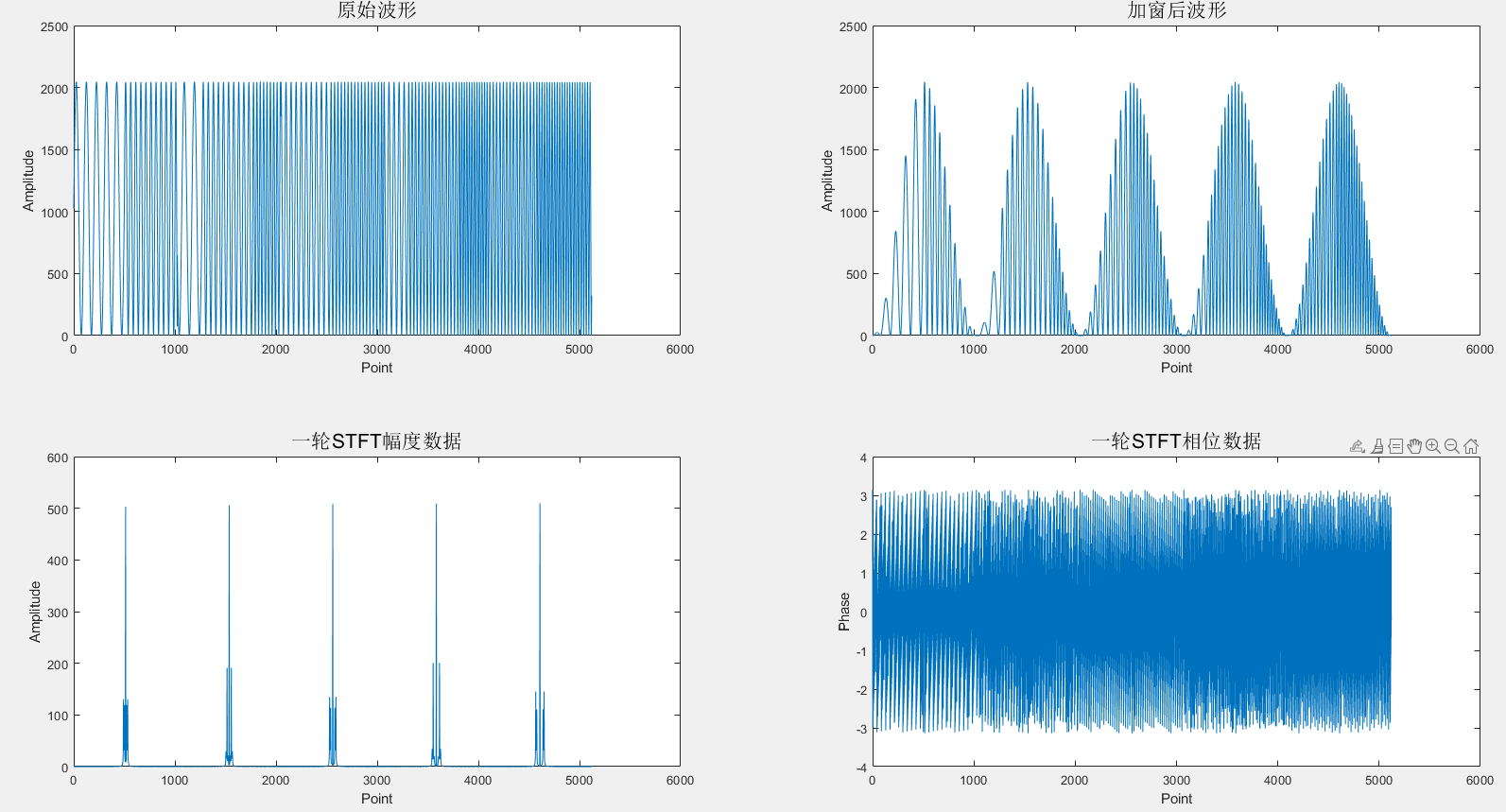展开全文• 多种短时傅里叶变换实现及其对比分析的程序，matlab源代码！附带文档进行说明！
•   在Matlab中，做短时傅里叶变换需要使用函数spectrogram，而在Matlab2019中，引入了一个新的函数stft，下面我们就来看下这两个函数都如何使用。   短时傅里叶变换的基本原理就是将数据分段加窗，做fft，在分段...
  在Matlab中，做短时傅里叶变换需要使用函数spectrogram，而在Matlab2019中，引入了一个新的函数stft，下面我们就来看下这两个函数都如何使用。
短时傅里叶变换的基本原理就是将数据分段加窗，做fft，在分段时会有overlap，因此一个向量的短时傅里叶变换结果是一个矩阵。了解了这点，下面的函数及参数就更加容易理解了。
spectrogram
参数列表
先来看spectrogram函数，在更早期的版本中，这个函数的名字是specgram，几种常用的用法如下：
spectrogram(x)
s = spectrogram(x)
s = spectrogram(x, window)
s = spectrogram(x, window, noverlap)
s = spectrogram(x, window, noverlap, nfft)
s = spectrogram(x, window, noverlap, nfft, fs)
[s, f, t] = spectrogram(x, window, noverlap, nfft, fs)
[s, f, t] = spectrogram(x, window, noverlap, f, fs)
[s, f, t, p] = spectrogram(x, window, noverlap, f, fs)

其中，
x表示输入信号；window表示窗函数，如果window的值是一个整数，那么被分段的x的每一段的长度都等于window，并采用默认的Hamming窗；如果window是一个向量，那么被分段后每一段的长度都等于length(window)，且输入的向量即为所要加的窗函数；overlap表示两段之间的重合点数，overlap的值必须要小于窗长，如果没有指定overlap，默认是窗长的一半，即50%的overlap；nfft表示fft的点数，fft的点数跟窗长可以是不同的，当没有指定该参数时，Matlab会取max(256, 2^(ceil(log2(length(window)))))，即当窗长小于256时，fft的点数是256；当窗长大于256时，fft的点数取大于窗长的最小的2的整数次幂；fs表示采样率，用来归一化显示使用；f表示显示的频谱范围，f是一个向量，长度跟s的行数相同；
当x是实信号且nfft为偶数时，s的行数为(nfft/2+1)当x是实信号且nfft为奇数时，s的行数为(nfft+1)/2当x是复信号时，s的行数为nfft当在输入的参数列表中指定f后，函数会在f指定的频率处计算频谱图，返回的f跟输入的f是相同的； t表示显示的时间范围，是一个向量，长度跟s的列数相同；p表示功率谱密度，对于实信号，p是各段PSD的单边周期估计；对于复信号，当指定F频率向量时，P为双边PSD;如何计算PSD
Examples
首先，生成信号如下，4个点频信号拼接起来：
clc;clear all;close all;
fs = 10e6;
n = 10000;
f1 = 10e3; f2 = 50e3; f3 = 80e3; f4 = 100e3;
t = (0:n-1)'/fs;
sig1 = cos(2*pi*f1*t);
sig2 = cos(2*pi*f2*t);
sig3 = cos(2*pi*f3*t);
sig4 = cos(2*pi*f4*t);

sig = [sig1; sig2; sig3; sig4];

信号的时域波形如下：直接调用spectrogram(sig)，可得如下结果，图中默认横轴是频率，纵轴是时间为了绘图更灵活，我们不直接用spectrogram绘图，而且求出s后，再对s单独绘图，这次我们指定window的大小为256
s = spectrogram(sig, 256);
t = linspace(0, 4*n/fs, size(s,1));
f = linspace(0, fs/2, size(s,2));
figure;
imagesc(t, f, 20*log10((abs(s))));xlabel('Samples'); ylabel('Freqency');
colorbar;noverlap默认是50%，现在我们把它设为window的长度减1，即每次的步进为1
s = spectrogram(sig, 256, 255);
t = linspace(0, 4*n/fs, size(s,1));
f = linspace(0, fs/2, size(s,2));
figure;
imagesc(t, f, 20*log10((abs(s))));xlabel('Samples'); ylabel('Freqency');
colorbar;再加上nfft和fs参数，我们指定fft点数就是窗长
s = spectrogram(sig, 256, 128, 256, fs);

这个的图形跟之前一样，不再画了

如果在返回值中，增加f和t，这样我们下面就不用再重新定义f和t了
[s, f, t] = spectrogram(sig, 256, 128, 256, fs);
figure;
imagesc(t, f, 20*log10((abs(s))));xlabel('Samples'); ylabel('Freqency');
colorbar;

从上面的图中我们可以看出，我们的4个信号的频率都比较小，而画出来的图显示的频谱范围比较大，导致下面很大一部分信息我们其实都不需要。这时，我们就可以通过指定f的区间来计算频谱。为了显示效果更好，我们把其他参数也调一下
window = 2048;
noverlap = window/2;
f_len = window/2 + 1;
f = linspace(0, 150e3, f_len);
[s, f, t] = spectrogram(sig, window, noverlap , f, fs);
figure;
imagesc(t, f, 20*log10((abs(s))));xlabel('Samples'); ylabel('Freqency');
colorbar;最后再把功率谱密度的返回值加上
[s, f, t, p] = spectrogram(sig, window, nfft, f, fs);
figure;
imagesc(t, f, p);xlabel('Samples'); ylabel('Freqency');
colorbar;stft
这个函数在Matlab的解释并不是很多，example也只写了两个，但用法比较简单：
window = 2048;
noverlap = window/2;
nfft = window;
[s, f, t, p] = spectrogram(sig, window, noverlap, nfft, fs);
figure;
imagesc(t, f, 20*log10((abs(s))));xlabel('Samples'); ylabel('Freqency');
title('使用spectrogram画出的短时傅里叶变换图形');
colorbar;

ss = stft(sig,fs,'Window',hamming(window),'OverlapLength',window/2,'FFTLength',nfft);
figure;
imagesc(t, f, 20*log10((abs(ss(1024:end,:)))));xlabel('Samples'); ylabel('Freqency');
title('使用stft画出的短时傅里叶变换图形');
colorbar;欢迎关注微信公众号：Qunat_Times
欢迎大家学习我的课程： System Generator & HLS数字信号处理教程展开全文stft 信号处理
• 目前网络关于分数阶傅里叶变换可靠的代码来源主要有一下几个： （1）来源一： M.A. Kutay. fracF: Fast computation of the fractional Fourier transform, 1996. www.ee.bilkent.edu.tr/~haldun/fracF.m. （2）...
• 短时傅里叶变换原理及其MATLAB实现（Short Time Fourier Transform，STFT） 1.短时Fourier变换原理（STFT原理） 信号x(t)短时Fourier变换定义为： 其中w(τ)为窗函数。 X(ω,t)中的时间t表示窗函数w(τ−t)的位置，...
短时傅里叶变换原理及其MATLAB实现（Short Time Fourier Transform，STFT）
1.短时Fourier变换原理（STFT原理）
信号x(t)短时Fourier变换定义为：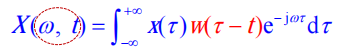其中w(τ)为窗函数。
X(ω,t)中的时间t表示窗函数w(τ−t)的位置，随着窗函数在整个区间上的滑动，可获得信号x(τ)在 t 附近区域上对应的频谱。
信号短时Fourier变换是一种常用的信号时频分析方法。
2.DFT中的STFT原理
信号x(t)的STFT是一个积分运算，在实际计算中也可通过DFT来实现，即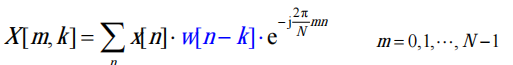其中：窗函数w[k]的宽度为N，x[k]为连续信号x(t)的抽样。
若抽样频率为fs，则存在 t=kT, T=1/fs。
3.时间分辨率和频谱分辨率 （2）时间分辨率 时间分辨率由时窗宽度Tp决定，公式为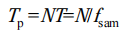Tp越小，时间分辨率越高。
（2）频谱分辨率 频谱分辨率是指分辩信号中相邻谱峰的能力，公式为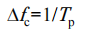∆fc越小，频谱分辨率越高。
4.不足 信号的STFT虽然能在一定程度上改善Fourier变换的不足，实现信号的时频分析，但其时间分辨率固定不变，因而不能有效地反映信号的突变程度，其应用受到局限。
小波分析拓展了信号STFT，实现了一种新的时频分析方法，其时窗可以随着信号的频率增高而缩小，频率降低而增大，有效地解决信号短时Fourier变换的缺陷，因而得到广泛应用。
5.Matlab代码实现
clear;
clc;

Fs=1e4;

t=0:1/Fs:4;
t1=0:1/Fs:1;

%10Hz的信号
x1=[sin(2*pi*10*t1)';zeros(Fs*3,1)];
%1000Hz的信号
x2=[zeros(Fs,1);sin(2*pi*1000*t1)';zeros(Fs*2,1)];
%2000Hz的信号
x3=[zeros(Fs*2,1);sin(2*pi*2000*t1)';zeros(Fs,1)];
%3000Hz的信号
x4=[zeros(Fs*3,1);sin(2*pi*3000*t1)'];
figure(1);
subplot(411);
plot(t,x1);
subplot(412);
plot(t,x2);
subplot(413);
plot(t,x3);
subplot(414);
plot(t,x4);

x=x1+x2+x3+x4;
figure(2);
subplot(211);
plot(t,x);
title('时域图');
axis([0 4 -2 2]);

%FFT代码
X=fft(x);
N=length(x);
f=(1:N/2)*Fs/N;
subplot(212);
plot(f,abs(X(1:N/2)));
xlabel('f (Hz)');
ylabel('|X(f)|');
title('频谱图');

%STFT关键代码
figure(3);
spectrogram(x,128,120,128,Fs,'yaxis');
title('时频图');

展开全文• 离散傅里叶变换使用的是一种全局变换，因为它表示一段时间内平均的频率特性，无法表述信号的时域局域性质，为了能够分析处理非平稳信号，人们对离散傅里叶变换进行了推广，提出了短时傅里叶变换。表达式如下所示： ...
• 根据一组程数据，在matlab中通过傅里叶变换求解频率f，并且画出相应的图形，振幅随频率变化的曲线等。
• matlab实现短时傅里叶变换的程序源码，自带例子，十分简单易懂，可以做频谱分析。
• matlab中不使用函数，根据短时傅里叶变换公式及定义，对输入的正弦信号手动实现STFT，画出频谱图等STFT...

# 短时傅里叶变换matlabmatlab 订阅